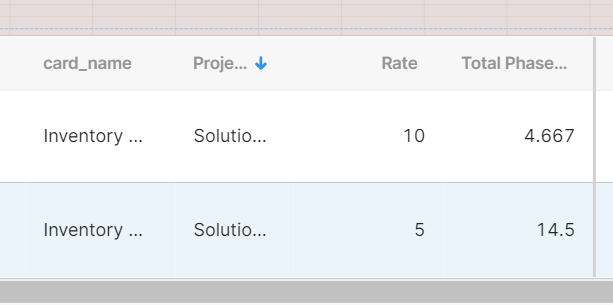# Do an aggregate calculation for each row of a table

I have a table that has a "rate" column and a "total phase hours" column:In the image above there are two rows in my table.

So in this example, I want to multiply the rate column by the total phase hours column for each row and then add the total row values together.

In the example this would look like (10 * 4.667) + (5 * 14.5) as the equation.

Is this possible to achieve without using a transformer?

I am able to get the addition of a column in each row by using this equation:
tableHours.data.TotalPhaseHours.reduce((x,y)=>x+y)

but I am confused by this specific use case.

Where do you want to store this information? in the same table or somewhere else?

Hey @David57940,

If you don't want to store (commit) that value against a specific record (What @ScottR is asking) you can solve the first part by adding a "Custom Column" to your table for a visual clue.

Set the column's value to:

``````currentRow.rate * currentRow.total_phase_hours
``````

Judging from your syntax (`tableHours.data.TotalPhaseHours`) your query is returning an Object of Arrays. It would be easier if it were an Array of Objects, so you can multiply each individual row correctly and sum it up.

That would look something like this:

``````formatDataAsArray(tableHours.data).reduce(function(p, c) {
return p + (c.rate * c.total_phase_hours);
}, 0);
``````

Does that work for you?

2 Likes

Thank you, this is exactly what I was looking for!

1 Like